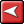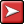## (TD) 1.16 Summary## (TD) 1.16 SummaryCongratulations! You have completed the Basic Drawing and Timing Analysis tutorial. In this tutorial we covered how to create a timing diagram, drawing simple signals and clocks, basic timing analysis with delays and setups, and advanced drawing techniques using equations and buses.What to do next:

If you will be doing lots of timing analysis, you need to read Section 5.1 Delays in the Timing Diagram Editor menu to find out about delay correlation and how delay times are calculated.

If you will need to make timing diagram documentation, then do the Display and Documentation tutorial.

If you are purchasing WaveFormer Pro or Data Sheet Pro, then do the Simulated Signals tutorial to discover the fastest way to generate timing diagrams.

If you are working with analog signals, then do the Analog Signals tutorial.

If you are going to be working with lots of timing parameters, do the Parameter Libraries tutorial to learn about making libraries.